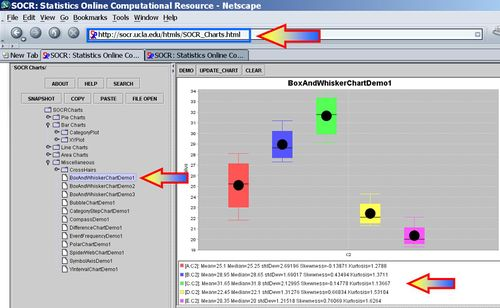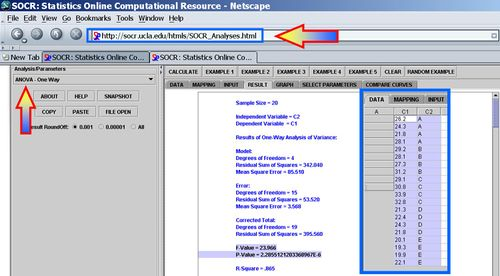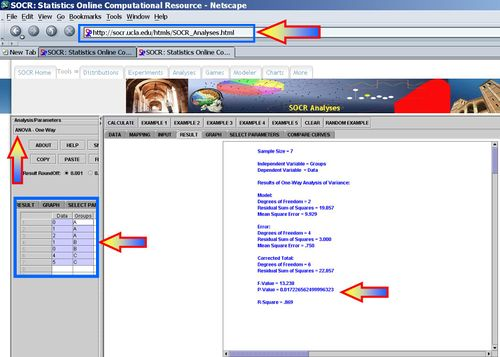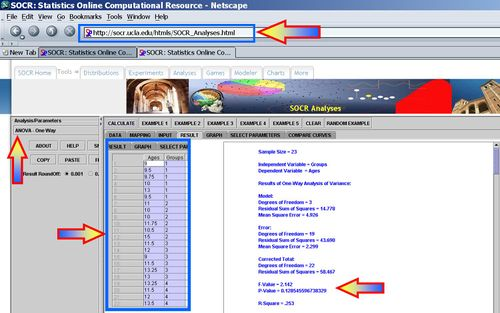# AP Statistics Curriculum 2007 ANOVA 1Way

## General Advance-Placement (AP) Statistics Curriculum - One-Way Analysis of Variance (ANOVA)

In the two-sample inference chapter, we considered the comparisons of two independent group means using the Independent T-test. Now, we expand our inference methods to study and compare k independent samples. In this case, we will be decomposing the entire variation in the data into (independent/orthogonal) components - i.e., we'll be analyzing the variance of the data. Hence, this procedure called Analysis of Variance (ANOVA).

### Motivational Example

Suppose 5 varieties of peas are currently being tested by a large agribusiness cooperative to determine which is best suited for production. A field was divided into 20 plots, with each variety of peas planted in four plots. The yields (in bushels of peas) produced from each plot are shown in two identical forms in the tables below.

 Variety of Pea A B C D E 26.2 29.2 29.1 21.3 20.1 24.3 28.1 30.8 22.4 19.3 21.8 27.3 33.9 24.3 19.9 28.1 31.2 32.8 21.8 22.1

 A 26.2,24.3,21.8,28.1 B 29.2,28.1,27.3,31.2 C 29.1,30.8,33.9,32.8 D 21.3,22.4,24.3,21.8 E 20.1,19.3,19.9,22.1

Using the SOCR Charts (see SOCR Box-and-Whisker Plot Activity and Dot Plot Activity), we can generate plots that enable us to compare visually the yields of the 5 different types peas.Using ANOVA, the data are regarded as random samples from k populations. Suppose the population means of the samples are $$\mu_1, \mu_2, \mu_3, \mu_4, \mu_5$$ and their population standard deviations are$\sigma_1, \sigma_2, \sigma_3, \sigma_4, \sigma_5$. We have 5 group means to compare. Why not just carry out $${5\choose 2}=10$$ T-tests comparing all (independent) pairs of groups?

Repeated T-tests would mean testing null hypotheses of the type $$H_o: \mu_i = \mu_j, \forall i\not= j$$. What is the problem with this approach? Suppose each test is carried out at $$\alpha = 0.05$$, so a type I error is 5% for each test. Then, the overall risk of a type I error is larger than 0.05 and gets much larger as the number of groups (k) gets larger. To solve this problem, we need to make multiple comparisons with an overall error of $$\alpha = 0.05$$ (or whichever level is specified initially).

The main idea behind ANOVA is that we need to know how much inherent variability exists is in the data before we can judge whether there is a difference in the sample means - i.e., presence of a grouping effect. To make an inference about means we compare two types of variability:

Variability between sample means
Variability within each group

It is very important that we keep these two types of variability in mind as we work through the following formulas. It is our goal to come up with a numerical recipe that describes/computes each of these variabilities.

### One-Way ANOVA Calculations

Let's make the following notation: $y_{i,j}$ = the measurement from group i, observation-index j.

k = number of groups

$n_i$ = number of observations in group i

n = total number of observations, $$n= n_1 + n_2 + \cdots + n_k$$
The group mean for group i is$\bar{y}_{i,.} = {\sum_{j=1}^{n_i}{y_{i,j}} \over n_i}$
The grand mean is$\bar{y}=\bar{y}_{.,.} = {\sum_{i=1}^k {\sum_{j=1}^{n_i}{y_{i,j}}} \over n}$

To compute the difference between the means, we will compare each group mean to the grand mean.

• SST (Sum Square due to Treatment, or Between Group variation): First, we describe the variation between the group means. For the independent T-test we described the difference between two group means as $$\bar{y_1} - \bar{y_1}$$. In ANOVA we describe the difference between k means as sums of squares due to treatments (or between-group variance):
SST(Between) = $$\sum_{i=1}^{k}{n_i(\bar{y}_{i,.}-\bar{y})^2}$$. SST can be thought of as the difference between each group mean and the grand mean.
Degrees of Freedom: df (Between) = k – 1.
Mean Sum Square due to Treatment (Between)$MST(Between) = {SST(Between)\over df(Between)}.$ This measures variability between the sample means.
• SSE (Sum Square due to Error, or Within Group Variation): Second, we assess the within group variation. Recall that to measure the variability within a single sample we used$\sqrt{\sum_{i=1}^n{(y_i - \bar{y})^2} \over n-1}$. In ANOVA to describe the combined variation within the k groups we use sums of squares due to error (within-group variation):
SSE(Within) = $$\sum_{i=1}^{k}{\sum_{j=1}^{n_i}{(y_{i,j}-\bar{y}_{i,.})^2}}$$, which can be thought of as the combination of variation within the k groups.
SSE(within)degrees of freedom: df (Within) = n - k
Mean variability within groups$MSE(Within) = {SSE(Within)\over df(Within)}.$ This is a measure of variability within the groups.
• Decomposition of Variance: Note that we have the following decomposition of the total variability in the data:
(Verbal): Deviation of an observation from the grand mean (Total variability) = Variation-Within + Variation_Between!
(Mathematics)$y_{i,j} - \bar{y} = (y_{i,j} - \bar{y}_{i,.}) + (\bar{y}_{i,.} - \bar{y})$. And summing up over all observations we get the desired ANOVA decomposition.
(ANOVA Decomposition)$\sum_{i=1}^{k}{\sum_{j=1}^{n_i}{(y_{i,j} - \bar{y})^2}} = \sum_{i=1}^{k}{\sum_{j=1}^{n_i}{(y_{i,j} - \bar{y}_{i,.})^2}} + \sum_{i=1}^{k}{n_i(\bar{y}_{i,.} - \bar{y})^2}$.
• Interpretations:
This means SST(total) = SSE(within) + SST(between)
df(Total) = df(Within) + df(Between) = (n - k) + (k – 1) = n - 1

### SOCR ANOVA Calculations

SOCR Analyses provide the tools to compute the 1-way ANOVA. For example, the ANOVA for the peas data above may be easily computed - see the image below. Note that SOCR ANOVA requires the data to be entered in this format:

 Variety of Pea Value Group-Index 26.2 A 24.3 A 21.8 A 28.1 A 29.2 B 28.1 B 27.3 B 31.2 B 29.1 C 30.8 C 33.9 C 32.8 C 21.3 D 22.4 D 24.3 D 21.8 D 20.1 E 19.3 E 19.9 E 22.1 EF-Value = 23.966
P-Value = 2.2855121203368967E-6

### ANOVA Table

 Variance Source Degrees of Freedom (df) Sum of Squares (SS) Mean Sum of Squares (MS) F-Statistics P-value Treatment Effect (Between Group) k-1 $$\sum_{i=1}^{k}{n_i(\bar{y}_{i,.}-\bar{y})^2}$$ $$MST(Between)={SST(Between)\over df(Between)}$$ $$F_o = {MST(Between)\over MSE(Within)}$$ $$P(F_{(df(Between), df(Within))} > F_o)$$ Error (Within Group) n-k $$\sum_{i=1}^{k}{\sum_{j=1}^{n_i}{(y_{i,j}-\bar{y}_{i,.})^2}}$$ $$MSE(Within)={SSE(Within)\over df(Within)}$$ F-Distribution Calculator Total n-1 $$\sum_{i=1}^{k}{\sum_{j=1}^{n_i}{(y_{i,j} - \bar{y})^2}}$$ ANOVA Activity

### ANOVA Hypotheses

The general form of the ANOVA hypotheses is: $H_o: \mu_1=\mu_2=\mu_3 = \cdots = \mu_k$ $H_a: \mu_i \not= \mu_j$ for some $$i\not=j$$.

Note that $$H_o$$ is compound hypothesis, when k > 2, so rejecting $$H_o$$ doesn't tell us which $$\mu_i$$'s are different. It only tells us that some two are not equal.

• Test Statistics: The test statistic$F_o = {MST(Between) \over MSE(Within)}$. If $$F_o$$ is large, then there is a lot of between group variation, relative to the within group variation. Therefore, the discrepancies between the group means are large compared to the variability within the groups (error). Hence, large values of $$F_o$$ provide strong evidence against $$H_o$$.

### Examples

#### Hands-on Example

This example is provided as a hands-on demonstration of the calculations performed in 1-way ANOVA. Clearly the value added by this example is conceptual understanding and appreciation of the methodological protocol for computing critical values and probabilities in ANOVA designs.

 Groups Index A B C 1 0 1 4 2 1 0 5 3 2 $$n_i$$ 2 3 2 s 1 3 9 $$\bar{y}_i$$ 0.5 1 4.5

Now use the ANOVA table to calculate the F-statistics and the corresponding p-value. Then use SOCR Analyses (ANOVA) to validate your manual calculations.

 Variance Source Degrees of Freedom (df) Sum of Squares (SS) Mean Sum of Squares (MS) F-Statistics P-value Treatment Effect (Between Group) 3-1 $$\sum_{i=1}^{k}{n_i(\bar{y}_{i,.}-\bar{y})^2}=19.86$$ $${SST(Between)\over df(Between)}={19.86\over 2}$$ $$F_o = {MST(Between)\over MSE(Within)}=13.24$$ $$P(F_{(df(Between), df(Within))} > F_o)=0.017$$ Error (Within Group) 7-3 $$\sum_{i=1}^{k}{\sum_{j=1}^{n_i}{(y_{i,j}-\bar{y}_{i,.})^2}}=3$$ $${SSE(Within)\over df(Within)}={3\over 4}$$ F-Distribution Calculator Total 7-1 $$\sum_{i=1}^{k}{\sum_{j=1}^{n_i}{(y_{i,j} - \bar{y})^2}}=22.86$$ Anova ActivityTherefore, in this case we can reject the null hypothesis (at $$\alpha = 0.05$$).

#### Walking Age

Parents are frequently concerned when their child seems slow to begin walking. In 1972, Science reported on an experiment in which the effects of several different treatments on the age at which a child’s first walks were compared. Children in the first group were given special walking exercises for 12 minutes daily beginning at the age 1 week and lasting 7 weeks. The second group of children received daily exercises, but not the walking exercises administered to the first group. The third and forth groups received no special treatment and differed only in that the third group’s progress was checked weekly and the forth was checked only at the end of the study. Observations on age (months) when the child began to walk are on the next slide.

 Age_(months) Treatment_Group 9 1 9.5 1 9.75 1 10 1 13 1 9.5 1 11 2 10 2 10 2 11.75 2 10.5 2 15 2 11.5 3 12 3 9 3 11.5 3 13.25 3 13 3 13.25 4 11.5 4 12 4 13.5 4 11.5 4• Conclusion: These data show that a child's true mean walking age is not statistically significantly different among any of the four treatment groups (p = 0.128546).

### ANOVA Conditions

ANOVA is valid if:

• Design conditions: All groups of observations represent random samples from their respective populations. Also required is that the samples, and observations within each group, are independent of one another.
• Population conditions: The k population distributions must be approximately Normal. Normality is less crucial if the sample sizes are large. Also it requires that the standard deviations of all populations are equal$\sigma_1=\sigma_2=\sigma_3=\cdots = \sigma_k$. This condition can be slightly relaxed in practice to $$0.5 \leq {\sigma_i \over \sigma_j} \leq 2$$, for all i and j, i.e., none of the population variances is twice larger than any of the other ones.

"-----